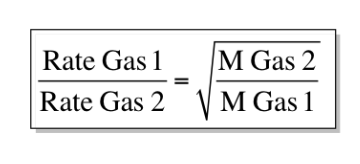# Problem: During the discussion of gaseous diffusion for enriching uranium, it was claimed that 235UF6 diffuses 0.4% faster than 238UF6. Show the calculation that supports this value. The molar mass of 235UF6 = 235.043930 + 6 × 18.998403 = 349.034348 g/mol, and the molar mass of 238UF6 = 238.050788 + 6 × 18.998403 = 352.041206 g/ mol.

🤓 Based on our data, we think this question is relevant for Professor Staff's class at NAU.

###### FREE Expert Solution

Using the Graham's Law of Effusion, we can calculate the approximate rate of each gases using their molar masses:###### Problem Details

During the discussion of gaseous diffusion for enriching uranium, it was claimed that 235UF6 diffuses 0.4% faster than 238UF6. Show the calculation that supports this value. The molar mass of 235UF6 = 235.043930 + 6 × 18.998403 = 349.034348 g/mol, and the molar mass of 238UF6 = 238.050788 + 6 × 18.998403 = 352.041206 g/ mol.